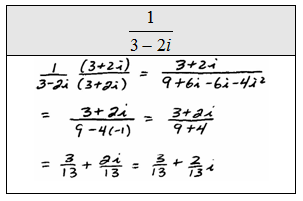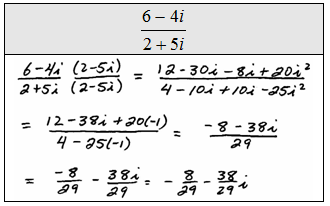## Algebra

Showing posts with label imaginary unit. Show all posts
Showing posts with label imaginary unit. Show all posts

### Complex Numbers

Up to this point, we have been working exclusively with real numbers.  Now we shed this limitation and allow for a much broader range of problems.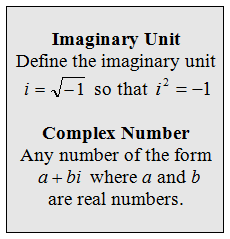With these definitions, we have greatly expanded our space of numbers.  Notice that any real number is also a complex number, for example 5 = 5 + 0i.  Here the real part is 5 and the imaginary part is 0.  Next we consider powers of i.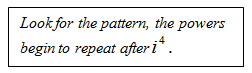Simplify.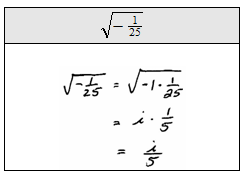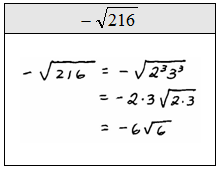Adding and subtracting complex numbers is just a matter of adding like terms. Be sure to use the order of operations and add real and imaginary parts separately.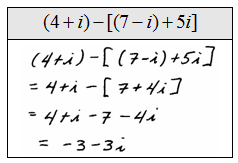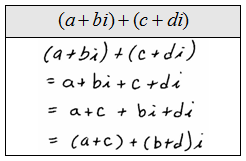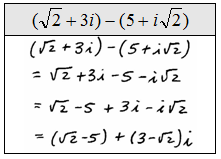We have to use a bit of caution when multiplying complex numbers.  First, we will run into i^2 often. In this case, we will replace them all with -1.  In addition, the property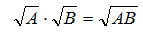is true only when either A or B is non-negative; therefore, simplify using the imaginary unit before multiplying.Tip: Make use of the imaginary unit if the radicand is negative before trying to simplify.

Multiply.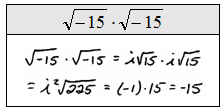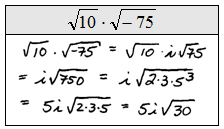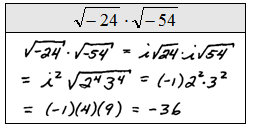Multiplying complex numbers often requires the distributive property.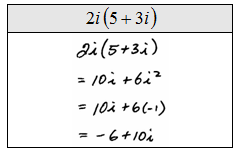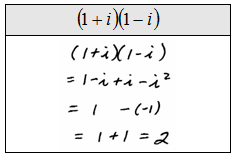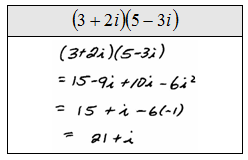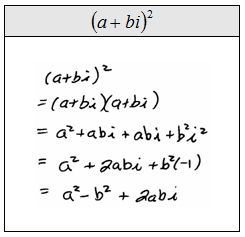Dividing complex numbers requires techniques similar to rationalizing the denominator.

Divide.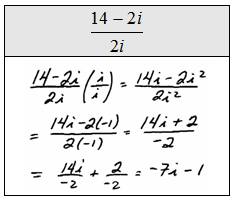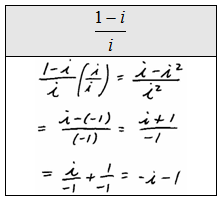When a complex number is in the denominator, multiply numerator and denominator by its conjugate.